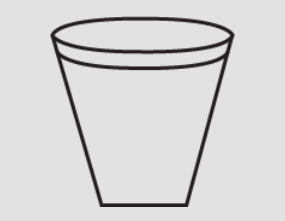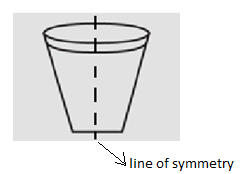Questions & AnswersQuestionAnswers

# Identify the shape given. Check whether it is symmetric or not. Draw the line of symmetry as well.Answer Verified
Hint:Draw a line of symmetry by cutting the shape into half.
Check if the 2 halves are the same, i.e. check if the figure is symmetric on both halves or not.

Complete step-by-step answer:
If a figure can be folded/ divided into half so that the two halves match each other exactly then such a figure is called a symmetric figure. In the figure, the dotted line divides the figure into two equal halves, forming the line of symmetry.
In general usage, symmetry most often refers to mirror or reflective symmetry, that is a line (in 2D) or a plane (in 3D) can be drawn through an object such that the two halves are mirror images of each other. An isosceles triangle and a human face are also some examples.
In the given figure, draw the line of symmetry.Hence, the given shape is symmetric as the line of symmetry divides it into two equal halves. The given shape is a frustum.

Note:The line of symmetry should pass through the center of the image/ shape to find the accurate symmetry.Such figures where the line of symmetry exists are called symmetrical figures.In certain fig there are 2 lines of symmetry.
Bookmark added to your notes.
View Notes
Figures with SymmetryConversion - One Shape to AnotherTwo Lines of SymmetryFactors of 6Table of 6 - Multiplication Table of 6CBSE Class 6 Maths Chapter 3 - Playing with Numbers FormulasLines of Symmetry in a ParallelogramLines of Symmetry in a RectangleSquare Root of 6CBSE Class 6 Maths Formulas